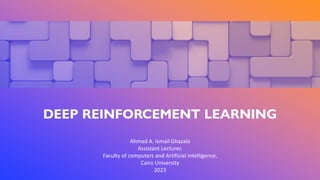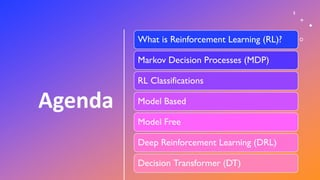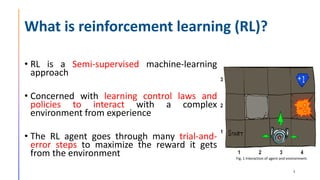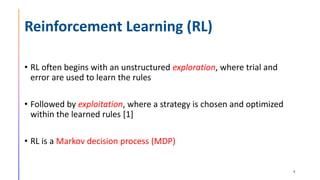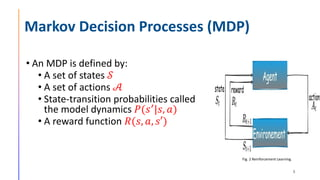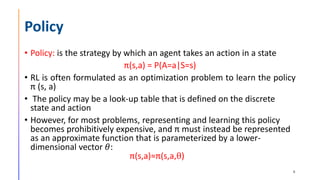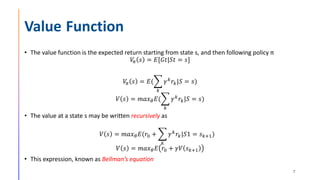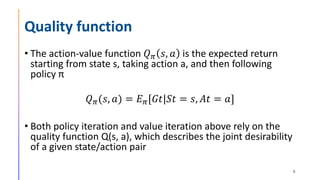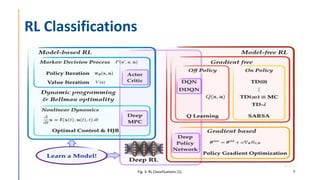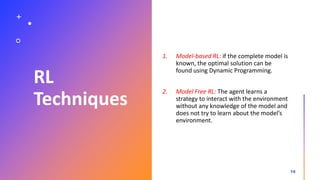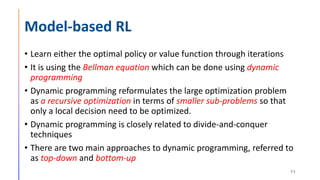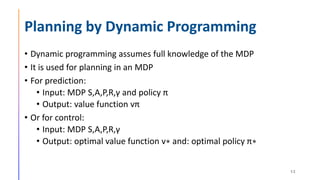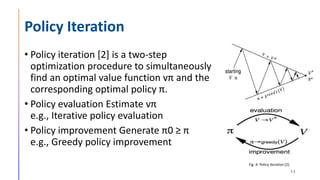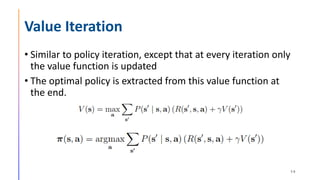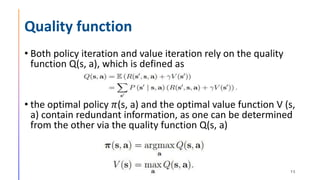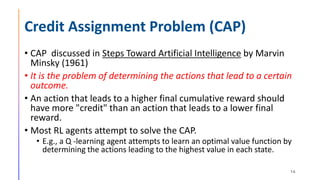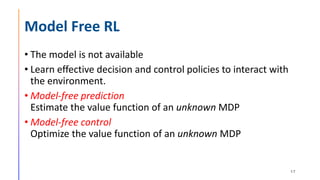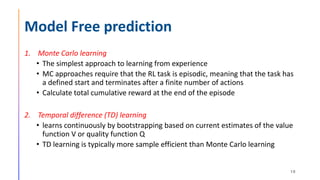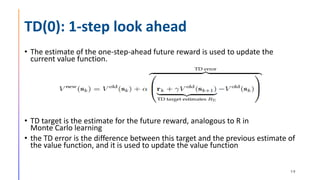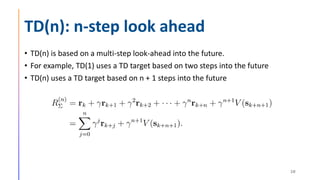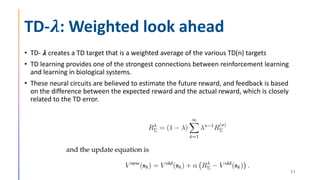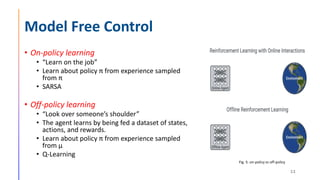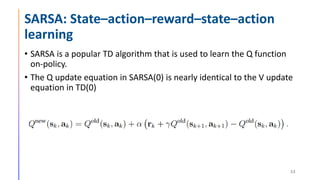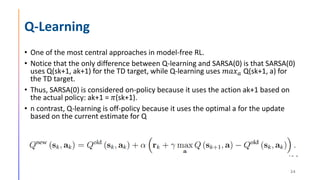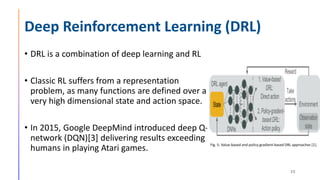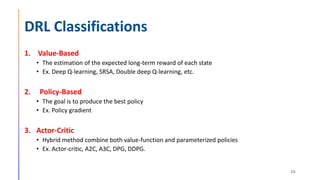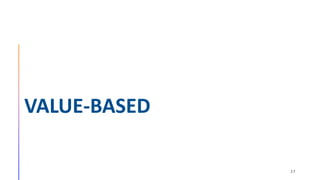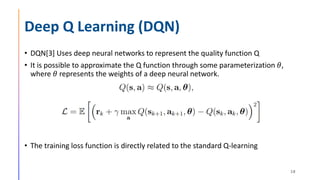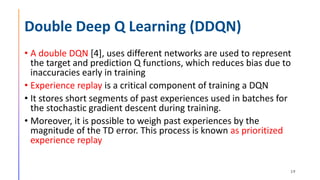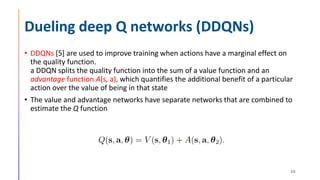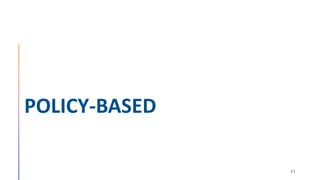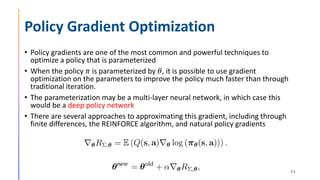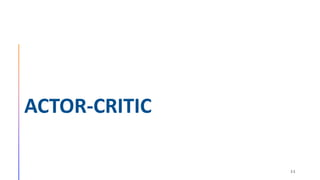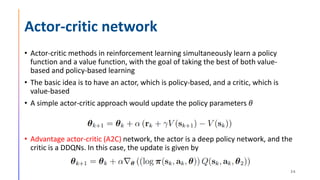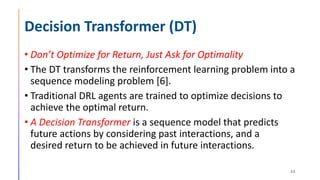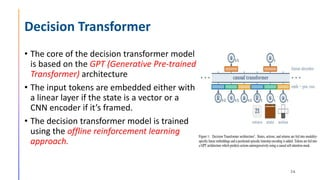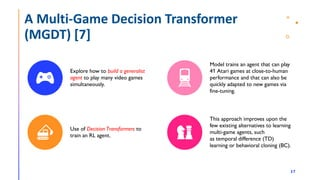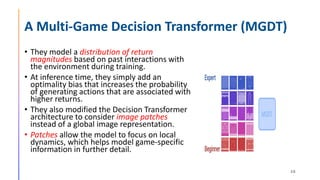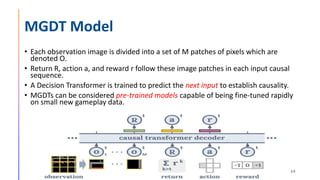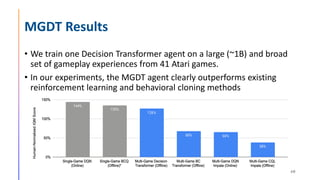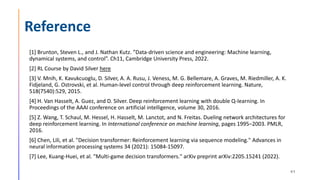1 of 42

### Deep Reinforcement learning

1. 1 DEEP REINFORCEMENT LEARNING Ahmad A. Ismail Ghazala Assistant Lecturer, Faculty of computers and Artificial Intelligence, Cairo University 2023
2. Agenda 2 What is Reinforcement Learning (RL)? Markov Decision Processes (MDP) RL Classifications Model Based Model Free Deep Reinforcement Learning (DRL) Decision Transformer (DT)
3. What is reinforcement learning (RL)? • RL is a Semi-supervised machine-learning approach • Concerned with learning control laws and policies to interact with a complex environment from experience • The RL agent goes through many trial-and- error steps to maximize the reward it gets from the environment 3 Fig. 3: Interaction of agent and environment. Fig. 1 Interaction of agent and environment.
4. Reinforcement Learning (RL) • RL often begins with an unstructured exploration, where trial and error are used to learn the rules • Followed by exploitation, where a strategy is chosen and optimized within the learned rules  • RL is a Markov decision process (MDP) 4
5. Markov Decision Processes (MDP) • An MDP is defined by: • A set of states 𝒮 • A set of actions 𝒜 • State-transition probabilities called the model dynamics 𝑃(𝑠′ |𝑠, 𝑎) • A reward function 𝑅(𝑠, 𝑎, 𝑠′) 5 Fig. 2 Reinforcement Learning.
6. Policy • Policy: is the strategy by which an agent takes an action in a state π(s,a) = P(A=a|S=s) • RL is often formulated as an optimization problem to learn the policy π (s, a) • The policy may be a look-up table that is defined on the discrete state and action • However, for most problems, representing and learning this policy becomes prohibitively expensive, and π must instead be represented as an approximate function that is parameterized by a lower- dimensional vector 𝜃: π(s,a)≈π(s,a,θ) 6
7. Value Function • The value function is the expected return starting from state s, and then following policy π 𝑉 𝜋 𝑠 = 𝐸[𝐺𝑡|𝑆𝑡 = 𝑠] 𝑉 𝜋 𝑠 = 𝐸(෍ 𝑘 𝛾𝑘𝑟𝑘|𝑆 = 𝑠) 𝑉 𝑠 = 𝑚𝑎𝑥𝜃𝐸(෍ 𝑘 𝛾𝑘𝑟𝑘|𝑆 = 𝑠) • The value at a state s may be written recursively as 𝑉 𝑠 = 𝑚𝑎𝑥𝜃𝐸(𝑟0 + ෍ 𝑘 𝛾𝑘𝑟𝑘|𝑆1 = 𝑠𝑘+1) 𝑉 𝑠 = 𝑚𝑎𝑥𝜃𝐸 𝑟0 + 𝛾𝑉 𝑠𝑘+1 • This expression, known as Bellman’s equation 7
8. Quality function • The action-value function 𝑄𝜋 𝑠, 𝑎 is the expected return starting from state s, taking action a, and then following policy π 𝑄𝜋(𝑠, 𝑎) = 𝐸𝜋[𝐺𝑡|𝑆𝑡 = 𝑠, 𝐴𝑡 = 𝑎] • Both policy iteration and value iteration above rely on the quality function Q(s, a), which describes the joint desirability of a given state/action pair 8
9. RL Classifications 9 Fig. 3: RL Classifications .
10. RL Techniques 1. Model-based RL: if the complete model is known, the optimal solution can be found using Dynamic Programming. 2. Model Free RL: The agent learns a strategy to interact with the environment without any knowledge of the model and does not try to learn about the model’s environment. 10
11. Model-based RL • Learn either the optimal policy or value function through iterations • It is using the Bellman equation which can be done using dynamic programming • Dynamic programming reformulates the large optimization problem as a recursive optimization in terms of smaller sub-problems so that only a local decision need to be optimized. • Dynamic programming is closely related to divide-and-conquer techniques • There are two main approaches to dynamic programming, referred to as top-down and bottom-up 11
12. Planning by Dynamic Programming • Dynamic programming assumes full knowledge of the MDP • It is used for planning in an MDP • For prediction: • Input: MDP S,A,P,R,γ and policy π • Output: value function vπ • Or for control: • Input: MDP S,A,P,R,γ • Output: optimal value function v∗ and: optimal policy π∗ 12
13. Policy Iteration • Policy iteration  is a two-step optimization procedure to simultaneously find an optimal value function vπ and the corresponding optimal policy π. • Policy evaluation Estimate vπ e.g., Iterative policy evaluation • Policy improvement Generate π0 ≥ π e.g., Greedy policy improvement 13 Fig. 4: Policy Iteration .
14. Value Iteration • Similar to policy iteration, except that at every iteration only the value function is updated • The optimal policy is extracted from this value function at the end. 14
15. Quality function • Both policy iteration and value iteration rely on the quality function Q(s, a), which is defined as • the optimal policy 𝜋(s, a) and the optimal value function V (s, a) contain redundant information, as one can be determined from the other via the quality function Q(s, a) 15
16. Credit Assignment Problem (CAP) • CAP discussed in Steps Toward Artificial Intelligence by Marvin Minsky (1961) • It is the problem of determining the actions that lead to a certain outcome. • An action that leads to a higher final cumulative reward should have more "credit" than an action that leads to a lower final reward. • Most RL agents attempt to solve the CAP. • E.g., a Q -learning agent attempts to learn an optimal value function by determining the actions leading to the highest value in each state. 16
17. Model Free RL • The model is not available • Learn effective decision and control policies to interact with the environment. • Model-free prediction Estimate the value function of an unknown MDP • Model-free control Optimize the value function of an unknown MDP 17
18. Model Free prediction 1. Monte Carlo learning • The simplest approach to learning from experience • MC approaches require that the RL task is episodic, meaning that the task has a defined start and terminates after a finite number of actions • Calculate total cumulative reward at the end of the episode 2. Temporal difference (TD) learning • learns continuously by bootstrapping based on current estimates of the value function V or quality function Q • TD learning is typically more sample efficient than Monte Carlo learning 18
19. TD(0): 1-step look ahead • The estimate of the one-step-ahead future reward is used to update the current value function. • TD target is the estimate for the future reward, analogous to R in Monte Carlo learning • the TD error is the difference between this target and the previous estimate of the value function, and it is used to update the value function 19
20. TD(n): n-step look ahead • TD(n) is based on a multi-step look-ahead into the future. • For example, TD(1) uses a TD target based on two steps into the future • TD(n) uses a TD target based on n + 1 steps into the future 20
21. TD-𝝀: Weighted look ahead • TD- 𝝀 creates a TD target that is a weighted average of the various TD(n) targets • TD learning provides one of the strongest connections between reinforcement learning and learning in biological systems. • These neural circuits are believed to estimate the future reward, and feedback is based on the difference between the expected reward and the actual reward, which is closely related to the TD error. 21
22. Model Free Control • On-policy learning • “Learn on the job” • Learn about policy π from experience sampled from π • SARSA • Off-policy learning • “Look over someone’s shoulder” • The agent learns by being fed a dataset of states, actions, and rewards. • Learn about policy π from experience sampled from µ • Q-Learning 22 Fig. 5: on-policy vs off-policy
23. SARSA: State–action–reward–state–action learning • SARSA is a popular TD algorithm that is used to learn the Q function on-policy. • The Q update equation in SARSA(0) is nearly identical to the V update equation in TD(0) 23
24. Q-Learning • One of the most central approaches in model-free RL. • Notice that the only difference between Q-learning and SARSA(0) is that SARSA(0) uses Q(sk+1, ak+1) for the TD target, while Q-learning uses 𝑚𝑎𝑥𝑎 Q(sk+1, a) for the TD target. • Thus, SARSA(0) is considered on-policy because it uses the action ak+1 based on the actual policy: ak+1 = 𝜋(sk+1). • n contrast, Q-learning is off-policy because it uses the optimal a for the update based on the current estimate for Q 24
25. Deep Reinforcement Learning (DRL) • DRL is a combination of deep learning and RL • Classic RL suffers from a representation problem, as many functions are defined over a very high dimensional state and action space. • In 2015, Google DeepMind introduced deep Q- network (DQN) delivering results exceeding humans in playing Atari games. 25 Fig. 5: Value-based and policy-gradient-based DRL approaches .
26. DRL Classifications 1. Value-Based • The estimation of the expected long-term reward of each state • Ex. Deep Q-learning, SRSA, Double deep Q-learning, etc. 2. Policy-Based • The goal is to produce the best policy • Ex. Policy gradient 3. Actor-Critic • Hybrid method combine both value-function and parameterized policies • Ex. Actor-critic, A2C, A3C, DPG, DDPG. 26
27. VALUE-BASED 27
28. Deep Q Learning (DQN) • DQN Uses deep neural networks to represent the quality function Q • It is possible to approximate the Q function through some parameterization 𝜃, where 𝜃 represents the weights of a deep neural network. • The training loss function is directly related to the standard Q-learning 28
29. Double Deep Q Learning (DDQN) • A double DQN , uses different networks are used to represent the target and prediction Q functions, which reduces bias due to inaccuracies early in training • Experience replay is a critical component of training a DQN • It stores short segments of past experiences used in batches for the stochastic gradient descent during training. • Moreover, it is possible to weigh past experiences by the magnitude of the TD error. This process is known as prioritized experience replay 29
30. Dueling deep Q networks (DDQNs) • DDQNs  are used to improve training when actions have a marginal effect on the quality function. a DDQN splits the quality function into the sum of a value function and an advantage function A(s, a), which quantifies the additional benefit of a particular action over the value of being in that state • The value and advantage networks have separate networks that are combined to estimate the Q function 30
31. POLICY-BASED 31
32. Policy Gradient Optimization • Policy gradients are one of the most common and powerful techniques to optimize a policy that is parameterized • When the policy 𝜋 is parameterized by 𝜃, it is possible to use gradient optimization on the parameters to improve the policy much faster than through traditional iteration. • The parameterization may be a multi-layer neural network, in which case this would be a deep policy network • There are several approaches to approximating this gradient, including through finite differences, the REINFORCE algorithm, and natural policy gradients 32
33. ACTOR-CRITIC 33
34. Actor-critic network • Actor-critic methods in reinforcement learning simultaneously learn a policy function and a value function, with the goal of taking the best of both value- based and policy-based learning • The basic idea is to have an actor, which is policy-based, and a critic, which is value-based • A simple actor-critic approach would update the policy parameters 𝜃 • Advantage actor-critic (A2C) network, the actor is a deep policy network, and the critic is a DDQNs. In this case, the update is given by 34
35. Decision Transformer (DT) • Don’t Optimize for Return, Just Ask for Optimality • The DT transforms the reinforcement learning problem into a sequence modeling problem . • Traditional DRL agents are trained to optimize decisions to achieve the optimal return. • A Decision Transformer is a sequence model that predicts future actions by considering past interactions, and a desired return to be achieved in future interactions. 35
36. Decision Transformer • The core of the decision transformer model is based on the GPT (Generative Pre-trained Transformer) architecture • The input tokens are embedded either with a linear layer if the state is a vector or a CNN encoder if it’s framed. • The decision transformer model is trained using the offline reinforcement learning approach. 36
37. A Multi-Game Decision Transformer (MGDT)  37 Explore how to build a generalist agent to play many video games simultaneously. Model trains an agent that can play 41 Atari games at close-to-human performance and that can also be quickly adapted to new games via fine-tuning. Use of Decision Transformers to train an RL agent. This approach improves upon the few existing alternatives to learning multi-game agents, such as temporal difference (TD) learning or behavioral cloning (BC).
38. A Multi-Game Decision Transformer (MGDT) • They model a distribution of return magnitudes based on past interactions with the environment during training. • At inference time, they simply add an optimality bias that increases the probability of generating actions that are associated with higher returns. • They also modified the Decision Transformer architecture to consider image patches instead of a global image representation. • Patches allow the model to focus on local dynamics, which helps model game-specific information in further detail. 38
39. MGDT Model • Each observation image is divided into a set of M patches of pixels which are denoted O. • Return R, action a, and reward r follow these image patches in each input causal sequence. • A Decision Transformer is trained to predict the next input to establish causality. • MGDTs can be considered pre-trained models capable of being fine-tuned rapidly on small new gameplay data. 39
40. MGDT Results • We train one Decision Transformer agent on a large (~1B) and broad set of gameplay experiences from 41 Atari games. • In our experiments, the MGDT agent clearly outperforms existing reinforcement learning and behavioral cloning methods 40
41. Reference  Brunton, Steven L., and J. Nathan Kutz. ”Data-driven science and engineering: Machine learning, dynamical systems, and control”. Ch11, Cambridge University Press, 2022.  RL Course by David Silver here  V. Mnih, K. Kavukcuoglu, D. Silver, A. A. Rusu, J. Veness, M. G. Bellemare, A. Graves, M. Riedmiller, A. K. Fidjeland, G. Ostrovski, et al. Human-level control through deep reinforcement learning. Nature, 518(7540):529, 2015.  H. Van Hasselt, A. Guez, and D. Silver. Deep reinforcement learning with double Q-learning. In Proceedings of the AAAI conference on artificial intelligence, volume 30, 2016.  Z. Wang, T. Schaul, M. Hessel, H. Hasselt, M. Lanctot, and N. Freitas. Dueling network architectures for deep reinforcement learning. In International conference on machine learning, pages 1995–2003. PMLR, 2016.  Chen, Lili, et al. "Decision transformer: Reinforcement learning via sequence modeling." Advances in neural information processing systems 34 (2021): 15084-15097.  Lee, Kuang-Huei, et al. "Multi-game decision transformers." arXiv preprint arXiv:2205.15241 (2022). 41
42. Thank You 42